Courses

# Probability Distribution (Part - 2) Mathematics Notes | EduRev

## Mathematics : Probability Distribution (Part - 2) Mathematics Notes | EduRev

The document Probability Distribution (Part - 2) Mathematics Notes | EduRev is a part of the Mathematics Course Additional Topics for IIT JAM Mathematics.
All you need of Mathematics at this link: Mathematics

Probability Distributions
A probability distribution is a mapping of all the possible values of a random variable to their corresponding probabilities for a given sample space.
The probability distribution is denoted as
P(X = x)
which can be written in short form as
P(x)
The probability distribution can also be referred to as a set of ordered pairs of outcomes and their probabilities. This is known as the probability function f(x).
This set of ordered pairs can be written as:
(x, f(x))
where the function is defined as:
(f(x) = P(X = x)
Cumulative Distribution Function (CDF)
The Cumulative Distribution Function (CDF) is defined as the probability that a random variable X with a given probability distribution f(x) will be found at a value less than x. The cumulative distribution function is a cumulative sum of the probabilities up to a given point.
The CDF is denoted by F(x) and is mathematically described as: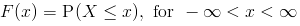Discrete Probability Distributions
Discrete random variables give rise to discrete probability distributions. For example, the probability of obtaining a certain number x when you toss a fair die is given by the probability distribution table below.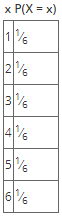For a discrete probability distribution, the set of ordered pairs (x,f(x)), where x is each outcome in a given sample space and f(x) is its probability, must follow the following:

• P(X = x) = f(x)
• f(x) ≥ 0
• ∑x f(x) = 1

Cumulative Distribution Function for a Discrete Random Variable For a discrete random variable, the CDF is given as follows: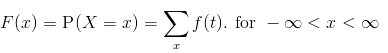In other words, to get the cumulative distribution function, you sum up all the probability distributions of all the outcomes less than or equal to the given variable.
For example, given a random variable X which is defined as the face that you obtain when you toss a fair die, find F(3)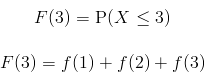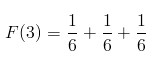F(3) = 1/2
The probability function can also found from the cumulative distribution function, for example f(3) = F(3) - F(2) - F(1) given that you know the full table of the cumulative distribution functions of the sample space.
Continuous Probability Distribution
Continuous random variables give rise to continuous probability distributions. Continuous probability distributions can't be tabulated since by definition the probability of any real number is zero i.e.
P(X = x) = 0
This is because the random variable X is continuous and as such can be infinitely divided into smaller parts such that the probability of selecting a real integer value x is zero.
Consequently, the continuous probability distribution is found as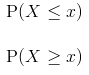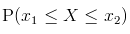and so on.
While a discrete probability distribution is characterized by its probability function (also known as the probability mass function), continuous probability distributions are characterized by their probability density functions.
Since we look at regions in which a given outcome is likely to occur, we define the Probability Density Function (PDF) as the a function that describes the probability that a given outcome will occur at a given point.
This can be mathematically represented as: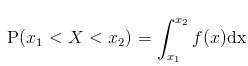In other words, the area under the curve.
For a continuous probability distribution, the set of ordered pairs (x,f(x)), where x is each outcome in a given sample space and f(x) is its probability, must follow the following: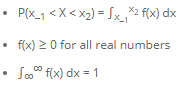Cumulative Distribution Function for a Continuous Probability Distribution

For a continuous random variable X, its CDF is given by
F(x) = P(X < x)
which is the same as saying: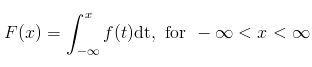and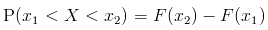From the above, we can see that to find the probability density function f(x) when given the cumulative distribution function F(x);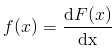if the derivative exists.
Continuous probability distributions are given in the form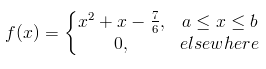whereby the above means that the probability density function f(x) exists within the region {x;a,b} but takes on the value of zero anywhere else.
For example, given the following probability density function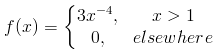Find

1. P(X ≤ 4)
2. P(X < 1)
3. P(2 ≤ X ≤ 3)
4. P(X > 1)
5. F(2)
Solutions:
1. P(X ≤ 4)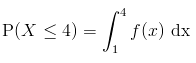Since we're finding the probability that the random variable is less than or equal to 4, we integrate the density function from the given lower limit (1) to the limit we're testing for (4).
We need not concern ourselves with the 0 part of the density function as all it indicates is that the function only exists within the given region and the probability of the random variable landing anywhere outside of that region will always be zero.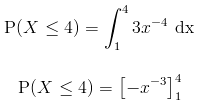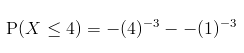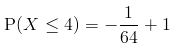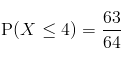2. P(X < 1)
P(X < 1) = 0 since the density function f(x) doesn't exist outside of the given boundary
3. P(2 ≤ X ≤ 3)
Since the region we're given lies within the boundary for which x is defined, we solve this problem as follows: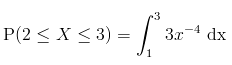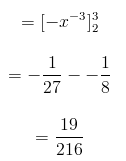4. P(X > 1)
The above problem is asking us to find the probability that the random variable lies at any point between 1 and positive Infinity. We can solve it as follows: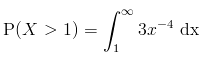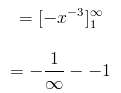but remember that we approximate the inverse of infinity to zero since it is too small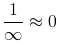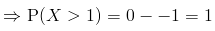The above is our expected result since we already defined f(x) as lying within that region hence the random variable will always be picked from there.
5. F(2)
The above is asking us to find the cumulative distribution function evaluated at 2.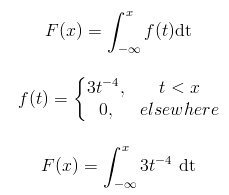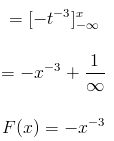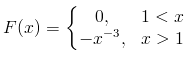Thus F(2) can be found from the above as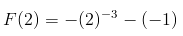F(2) = 7/8

Offer running on EduRev: Apply code STAYHOME200 to get INR 200 off on our premium plan EduRev Infinity!

40 docs

,

,

,

,

,

,

,

,

,

,

,

,

,

,

,

,

,

,

,

,

,

;